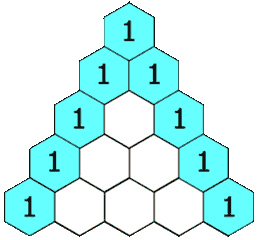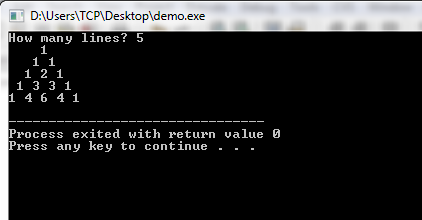# Program for Pascal Triangle in C and C++

Here I have shared simple program for pascal triangle in C and C++.

Basically Pascal’s triangle is a triangular array of binomial coefficients. An example for how pascal triangle is generated is illustrated in below image. If you have any doubts then you can ask it in comment section.

Each number is the sum of the two directly above it.## Program for Pascal Triangle in C++

Output### 6 thoughts on “Program for Pascal Triangle in C and C++”

1.good

2.good… can you post the code for pascal triangle in c#.net?

3.hey when i typed nos. of lines = 20
some negative nos. came whyy??

1.Check the second for loop

4.palindrome nos. are those nos. whose reverse is same ….crazy programmer is my program right … i mean can i get full marks for it in exam as it works.. |
|
v
see it
0)=================>
#include
int main()
{ using namespace std;
int a,b,c,j,f;
cout<<" enter a no. at hundred no."<<"\n"
<<"enter a no. at tense place "<<"\n"
<<"enter a nos. at unit place "<>a>>b>>c;
j=a*100+b*10+c;
cout<<" the entered nos. is "<< j<<"\n";
f=c*100+b*10+a;
cout<<" the reverse nos. is "<<f;
if(j==f)
{
cout<<"\n"<<" as we can see its palindrome number";
}
else
{
cout<<"\n"<<" as we can see it is not palindrome";
}
return 0;
}

5.instead of using alphabets as variables name use words it will make program easy to read